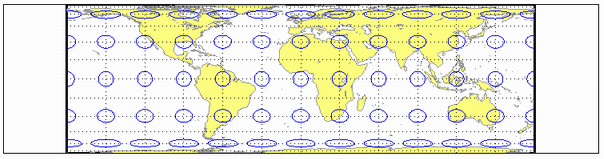# lambcyln

Lambert Equal-Area Cylindrical Projection

Cylindrical

## Identifier

`lambcyln`

## Graticule

Meridians: Equally spaced straight parallel lines 0.32 as long as the Equator.

Parallels: Unequally spaced straight parallel lines, perpendicular to the meridians. Spacing is closest near the poles.

Poles: Straight lines equal in length to the Equator.

Symmetry: About any meridian or the Equator.

## Features

This is an orthographic projection onto a cylinder tangent at the Equator. It is equal-area, but distortion of shape increases with distance from the Equator. Scale is true along the Equator and constant between two parallels equidistant from the Equator. This projection is not equidistant.

## Parallels

For cylindrical projections, only one standard parallel is specified. The other standard parallel is the same latitude with the opposite sign. For this projection, the standard parallel is by definition fixed at 0º.

## Remarks

This projection is named for Johann Heinrich Lambert and is a special form of the Equal-Area Cylindrical projection tangent at the Equator.

## Example

```landareas = shaperead('landareas.shp','UseGeoCoords',true); axesm ('lambcyln', 'Frame', 'on', 'Grid', 'on'); geoshow(landareas,'FaceColor',[1 1 .5],'EdgeColor',[.6 .6 .6]); tissot;```## Version History

Introduced before R2006a